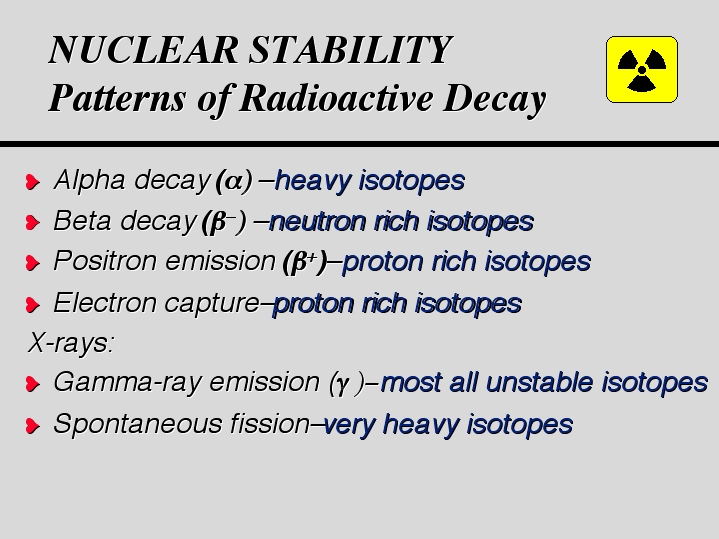How do isotopes become stable?

Jul 25, 2014

Most isotopes become stable by emitting alpha particles, beta particles, positrons, or gamma rays. A few become stable by electron capture or by spontaneous fission.

ALPHA PARTICLES

An alpha particle is a helium nucleus. The heavier elements usually decay by alpha emission. For example,

$\text{_92^238"U" → _90^234"Th" + _2^4"He}$

BETA PARTICLES

A beta particle is an electron. Nuclei that have too few protons can decay by converting a neutron to a proton and emitting a beta particle. For example,

₁₅³²P → ₁₆³²S + ₋₁⁰e

POSITRONS

A positron is the antimatter counterpart of an electron. Nuclei that have too many protons can decay by converting a proton to a neutron and emitting a positron. For example,

$\text{_5^8"B}$$\text{_4^8"Be}$ + $\text{_1^0"e}$

GAMMA RAYS:

Gamma rays are high-energy photons. When a nucleus emits an alpha or beta particle, the new nucleus may have excess energy. It can release this excess energy by emitting gamma rays.

Thus, thorium-234 becomes more stable by releasing gamma rays and a beta particle.

₉₀²³⁴Th → ₉₁²³⁴Pa + ₋₁⁰e + γ

ELECTRON CAPTURE

An isotope with too many protons in the nucleus may capture an electron. A proton becomes a neutron, so the atomic number decreases but the atomic mass stays the same.

An example of electron capture is the decay of beryllium-7.

₄⁷Be + ₋₁⁰e → ₃⁷Li

SPONTANEOUS FISSION

Nuclei with mass numbers greater than 230 often become stable by spontaneous fission.

For example

$\text{_98^252"Cf}$$\text{_54^140"Xe" " + " ""_44^108"Ru" " +" 4_0^1"n}$